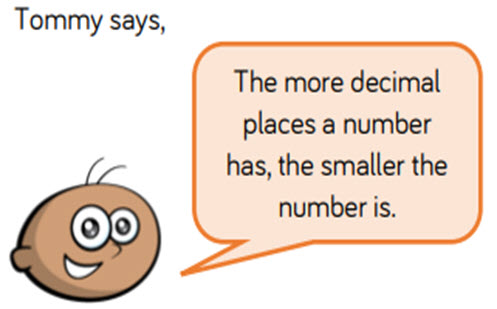# Decimals: multiplying and dividing decimal numbers

This guide provides a sequence of small steps for deepening understanding of decimal numbers, from understanding thousandths and numbers to three decimal places, to multiplying and dividing decimals by integers, and converting between fractions and decimals.

Year level(s) Year 5, Year 6
Audience Teacher
Purpose Teaching resource, Teaching strategies
Teaching strategies and pedagogical approaches Questioning, Explicit teaching
Keywords partition, integers, reasoning, problem-solving, explicit teaching

## Curriculum alignment

Curriculum connections Numeracy
Strand and focus Number, Build understanding
Topics Decimals, Fractions, Multiplication and division
AC: Mathematics (V9.0) content descriptions
AC9M5N01
Interpret, compare and order numbers with more than 2 decimal places, including numbers greater than one, using place value understanding; represent these on a number line

AC9M6N06
Multiply and divide decimals by multiples of powers of 10 without a calculator, applying knowledge of place value and proficiency with multiplication facts; using estimation and rounding to check the reasonableness of answers

Numeracy progression Number and place value (P8, P9)
Multiplicative strategies (P9)